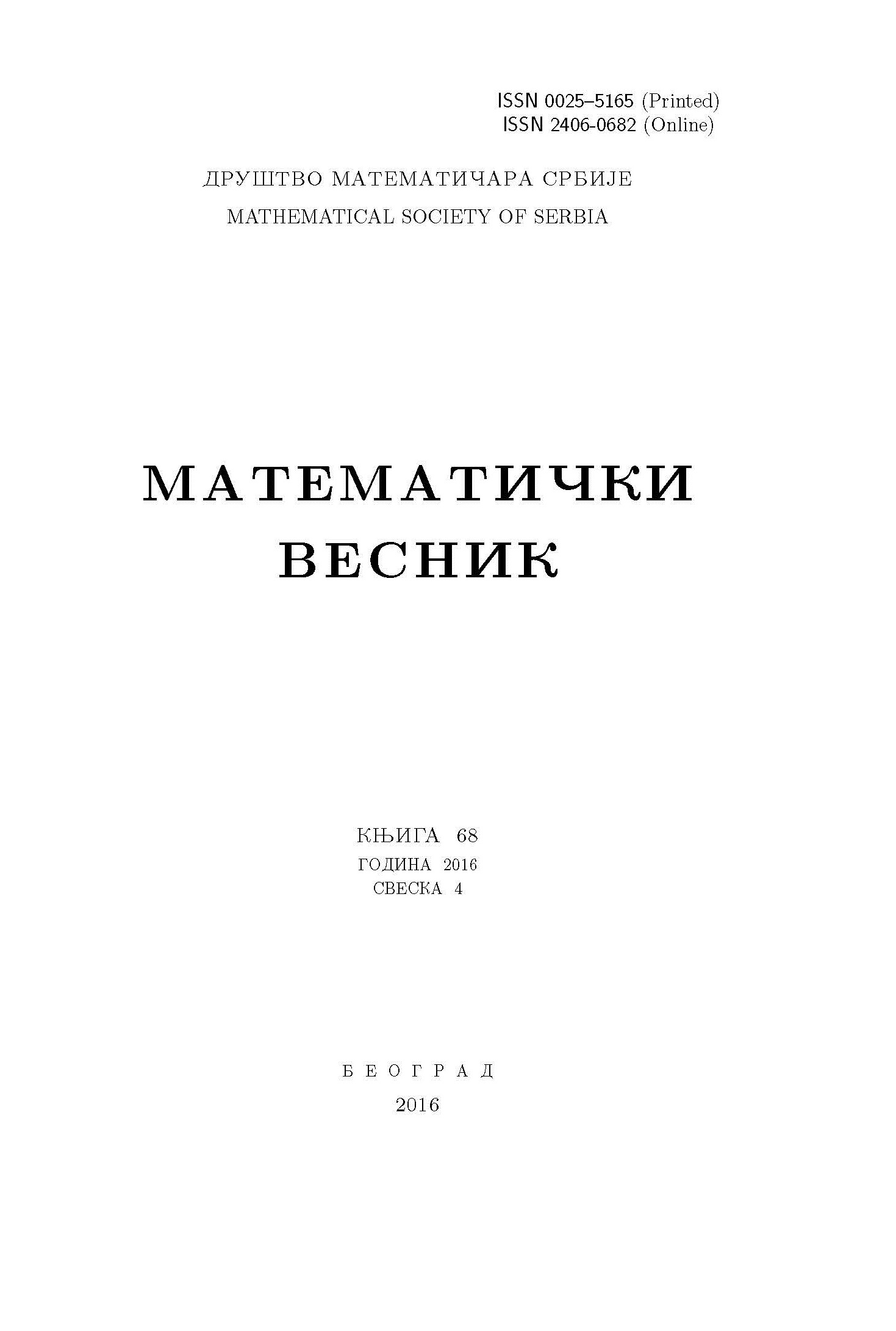﻿ Matematički Vesnik ﻿
 MATEMATIČKI VESNIK МАТЕМАТИЧКИ ВЕСНИКON THE GENERALIZED DISTANCE EIGENVALUES OF GRAPHS A. Alhevaz, M. Baghipur, H. A. Ganie, K. C. Das AbstractFor a simple connected graph $G$, the generalized distance matrix $D_{\alpha}(G)$ is defined as $D_{\alpha}(G)=\alpha Tr(G)+(1-\alpha)D(G)$, $0\leq \alpha\leq 1$. The largest eigenvalue of $D_{\alpha}(G)$ is called the generalized distance spectral radius or $D_{\alpha}$-spectral radius of $G$. In this paper, we obtain some upper bounds for the generalized distance spectral radius in terms of various graph parameters associated with the structure of graph $G$, and characterize the extremal graphs attaining these bounds. We determine the graphs with minimal generalized distance spectral radius among the trees with given diameter $d$ and among all unicyclic graphs with given girth. We also obtain the generalized distance spectrum of the square of the cycle and the square of the hypercube of dimension $n$. We show that the square of the hypercube of dimension $n$ has three distinct generalized distance eigenvalues.Keywords: Generalized distance matrix (spectrum); spectral radius; hypercube; unicyclic graph. MSC: 05C50, 05C12, 15A18 Pages:  1$-$14

﻿